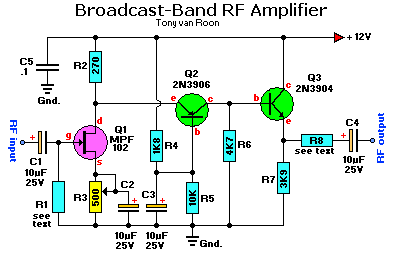# Broadcast-Band RF pojačalo

Broadcast-Band RF pojačaloParts List:
R1 = see text C1,C2,C3,C4 = 10uF, 25volt electrolytic
R2 = 270 ohms C5 = 0.1uF, ceramic disc
R3 = 500 ohm potentiometer
R4 = 1K8 (1800 ohms) Q1 = MPF102 J-FET, or use NTE451
R5 = 10K (10,000 ohms) Q2 = 2N3906, PNP-transistor (or use NTE159)
R6 = 4K7 (4700 ohms) Q3 = 2N3904, NPN-transistor (or use NTE123AP)
R7 = 3K9 (3900 ohms) see note about the ‘AP’ extension.

Izvor: tonys website

🔥325